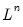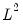Command Reference : Matrix Language Reference

 @norm
Norm of matrix.
Syntax: @norm(m[, n])
m: matrix, vector, sym, series
n: (optional) integer
Return: number
Returns the norm of m.
If no norm type is provided, this function returns the infinity norm. Possible choices for the norm type n include “–1” for the infinity norm, “0” for the Frobenius norm, and an integer “n” for thenorm.
Examples
matrix m1 = @mnrnd(10, 4)
scalar sc1 = @norm(m1)
computes the infinity norm of the matrix M1.
sym s1 = @inner(m1)
scalar sc2 = @norm(s1, 2)
computes thenorm of the symmetric matrix S1.
series x = nrnd
scalar sc3 = @norm(x)
assigns to SC3 the norm of the series X computed over the entire workfile.
Cross-references